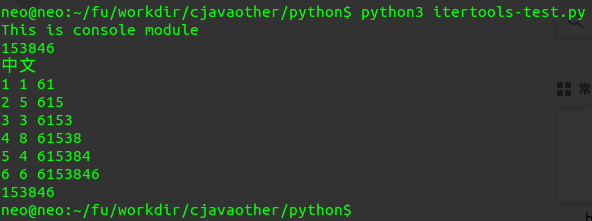# python -- 寻找最小正整数N，满足某个条件

#找出具有下列各性质的最小正整数 n：它的最后一位数字是6，如果把最后的6去掉并放在最前面所得到的数是原来数的4倍。

#-*-coding:utf8;-*-

# 第一种是网上的一种暴力解法：每次加10，然后判断是否符合条件
print("This is console module")
from itertools import count

for r in count(6,10):
#    print(r)
if int('6'+str(r)[:-1])/r==4.0:
print(r)
break
print('中文')

# 第二种，是我的思路，每次将div的最低位补到最后面，然后计算，知道mod为0就结束循环。
start = 6
end = start
mod = 1
count = 0
while mod != 0:
count += 1
div, mod = divmod(end, 4)
div = div % 10
end = end * 10 + div
print(count, div, end)
print(end-start*pow(10, count)) # 去掉最高位的start